# Balancing Chemical EquationsPage 1

#### WATCH ALL SLIDES

Slide 1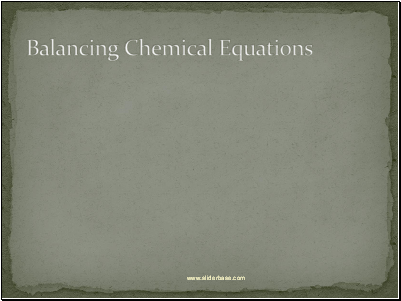Balancing Chemical Equations

Slide 2## What is a chemical equation?

Chemical equation - Describes a chemical change.

Parts of an equation:

Slide 3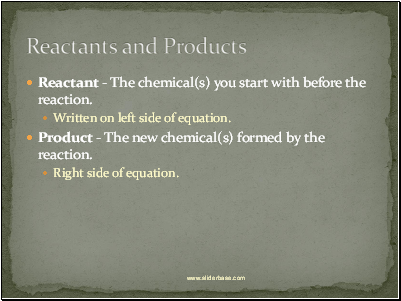## Reactants and Products

Written on left side of equation.

Product - The new chemical(s) formed by the reaction.

Right side of equation.

Slide 4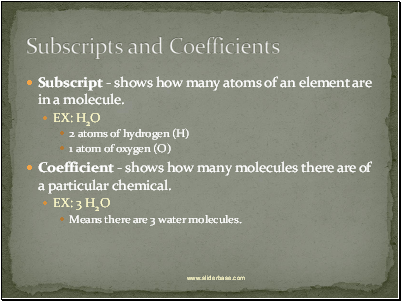## Subscripts and Coefficients

Subscript - shows how many atoms of an element are in a molecule.

EX: H2O

2 atoms of hydrogen (H)ю

1 atom of oxygen (O)ю

Coefficient - shows how many molecules there are of a particular chemical.

EX: 3 H2O

Means there are 3 water molecules.

Slide 52H2 + O2  2H2O

A Chemical Reaction

Slide 6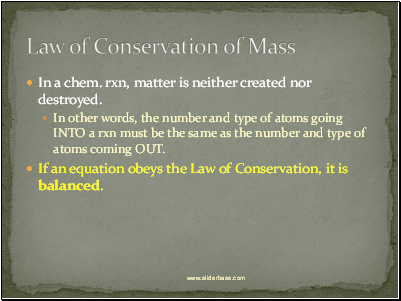## Law of Conservation of Mass

In a chem. rxn, matter is neither created nor destroyed.

In other words, the number and type of atoms going INTO a rxn must be the same as the number and type of atoms coming OUT.

If an equation obeys the Law of Conservation, it is balanced.

Slide 7## An Unbalanced Equation

CH4 + O2  CO2 + H2O

Reactant Side

Product Side

1 carbon atom

4 hydrogen atoms

2 oxygen atoms

1 carbon atom

2 hydrogen atoms

3 oxygen atoms

Slide 8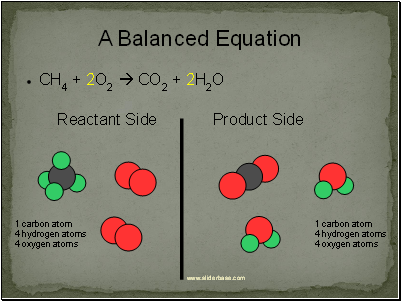## A Balanced Equation

CH4 + 2O2  CO2 + 2H2O

Reactant Side

Product Side

1 carbon atom

4 hydrogen atoms

4 oxygen atoms

1 carbon atom

4 hydrogen atoms

4 oxygen atoms

Slide 9## Rules of the Game

1. Matter cannot be created or destroyed.

2. Subscripts cannot be added, removed, or changed.

3. You can only change coefficients.

4. Coefficients can only go in front of chem. formulas .NEVER in the middle of a formula.

A few extra tips:

Try balancing big formulas first; save free elements for last.

If the same polyatomic ion appears on both sides of the equation, it’s usually okay to treat it as one unit.

There is no one particular way to balance equations. Some equations are harder to balance than others and might require some creativity to solve.

Slide 10

Go to page:
1  2